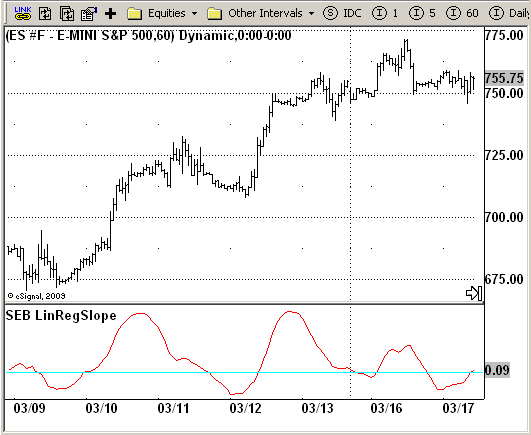# SEB Linear Regression Slope

ICE Data Services -

 SEB_LinRegSlope.efs EFSLibrary - Discussion Board

File Name: SEB_LinRegSlope.efs

Description:
This indicator plots the slope of linear regression in osccilator-like
manner. This is a part of Standard Error Bands study.

Formula Parameters:
Length : 21

Notes:
Linear Regression is a concept also know as the "least squares method" or
"best fit". Linear Regression attempts to fit a straight line between several
data points in such a way that distance between each data point and the line is
minimized.
"Standard Error Bands" Jon Anderson, Stocks&Commodities Magazine, Traders Tips, 09/1996`/*********************************Provided By: eSignal (Copyright c eSignal), a division of Interactive Data Corporation. 2009. All rights reserved. This sample eSignal Formula Script (EFS) is for educational purposes only and may be modified and saved under a new file name. eSignal is not responsible for the functionality once modified. eSignal reserves the right to modify and overwrite this EFS file with each new release.Description: This indicator plots the slope of linear regression in osccilator-like manner. This is a part of Standard Error Bands study. Version: 1.0 03/19/2009Formula Parameters: Default: Length 21 Notes: Linear Regression is a concept also know as the "least squares method" or "best fit". Linear Regression attempts to fit a straight line between several data points in such a way that distance between each data point and the line is minimized. "Standard Error Bands" Jon Anderson, Stocks&Commodities Magazine, Traders Tips, 09/1996 **********************************/var fpArray = new Array();var bInit = false;function preMain() { setStudyTitle("SEB LinRegSlope"); setCursorLabelName("Slope", 0); setDefaultBarFgColor(Color.red, 0); addBand(0, PS_SOLID, 1, Color.cyan); var x=0; fpArray[x] = new FunctionParameter("Length", FunctionParameter.NUMBER); with(fpArray[x++]){ setLowerLimit(1); setDefault(21); }}var xSL = null;function main(Length) {var nBarState = getBarState();var nSL = 0; if (nBarState == BARSTATE_ALLBARS) { if (Length == null) Length = 21; } if (bInit == false) { xSL = efsInternal("calc", Length); bInit = true; } nSL = xSL.getValue(0); if (nSL == null) return; return nSL;}var xClose = null;var xInit = false;function calc(Length) {var SL = 0;var SumBars = Length * (Length - 1) * 0.5;var SumSqrBars = (Length - 1) * Length * (2 * Length - 1) / 6;var Sum1 = 0;var SumY = 0;var i = 0; if(xInit==false){ xClose = close(); xInit = true; } for (i = 0; i < Length; i++) { Sum1 += i * xClose.getValue(-i); SumY += xClose.getValue(-i); } var Sum2 = SumBars * SumY; var Num1 = Length * Sum1 - Sum2; var Num2 = SumBars * SumBars - Length * SumSqrBars; SL = Num1 / Num2; return SL;}`